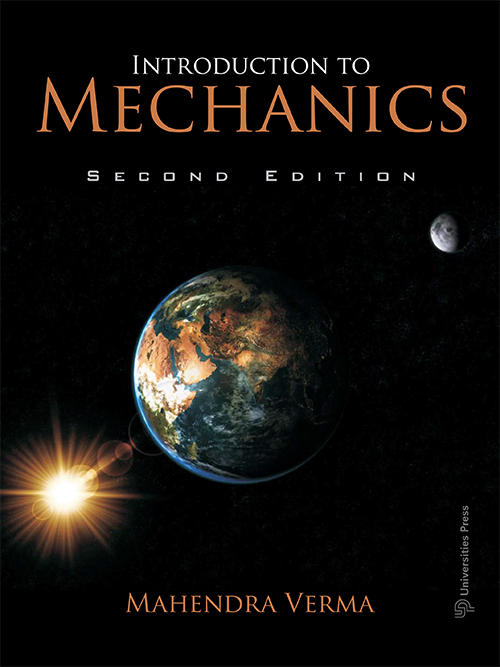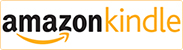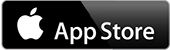## Introduction to Mechanics

#### Ebook available on :Introduction to Mechanics, Second Edition offers a modern introduction to Newtonian dynamics and the basics of special relativity. The present edition covers almost all the topics specified in the mechanics syllabus of most Indian universities. It preserves the emphasis laid on the fundamental principles of mechanics and introduction of modern topics (as in the earlier edition), such as symmetries, nonlinear dynamics and presentation of Newton's laws as a differential equation. The programming language Python is used to solve a large number of differential equations numerically and for many plots.

For computer programs, class PPTs, figures and discussions, please visit the author’s webpage. Link to author’s webpage: http://home.iitk.ac.in/~mkv/Mechanics-book/Welcome.html

#### Contributors (Author(s), Editor(s), Translator(s), Illustrator(s) etc.)

Mahendra Verma obtained his doctoral degree from the University of Maryland, College Park. He joined the Department of Physics, IIT Kanpur in 1994. He is a non-linear dynamist whose chief interest lies in theoretical and computational studies of turbulence and nonlinear physics. Currently he is working on magnetohydrodynamic turbulence, dynamo and convective turbulence. Dr Verma is also interested in atmospheric and computational physics. He is a recipient of the Swarnajayanti fellowship.

Preface to the Second Edition
Preface to the First Edition
Notation
1 History of mechanics
2 Newton’s laws of motion
3 Forces
4 Kinematics vs dynamics
5 Motion in one dimension
6 Numerical solution of newton’s equations
7 Phase space description of mechanical systems
8 Symmetry properties of newton’s equation
9 Two-dimensional motion; central force problem
10 Three-dimensional motion
11 Energy
12 Motion in a noninertial reference frame
13 Conservation of linear momentum and centre of mass
14 Collisions
15 Rotation dynamics: definitions
16 Rigid body dynamics
17 Nonlinear dynamics and chaos
18 Statics
19 Mechanics of solids
20 Mechanics of fluids
21 Special theory of relativity: kinematics
22 Relativistic dynamics
Epilogue
Appendix A: Present paradigm of physics and science
Appendix B: Dimensional analysis and estimation
Appendix C: Python programming language
Appendix D: Matlab, Scilab and Octave
Appendix E: Tensors and moment of inertia tensor
Appendix F: Vector operations on vector and scalar fields
Appendix G: Important astronomical data
Appendix H: Important physical constants
Appendix I: Hyperbolic functions
Appendix J: Torque-free precession revisited